# Tutorial: III-V Nanosheet FET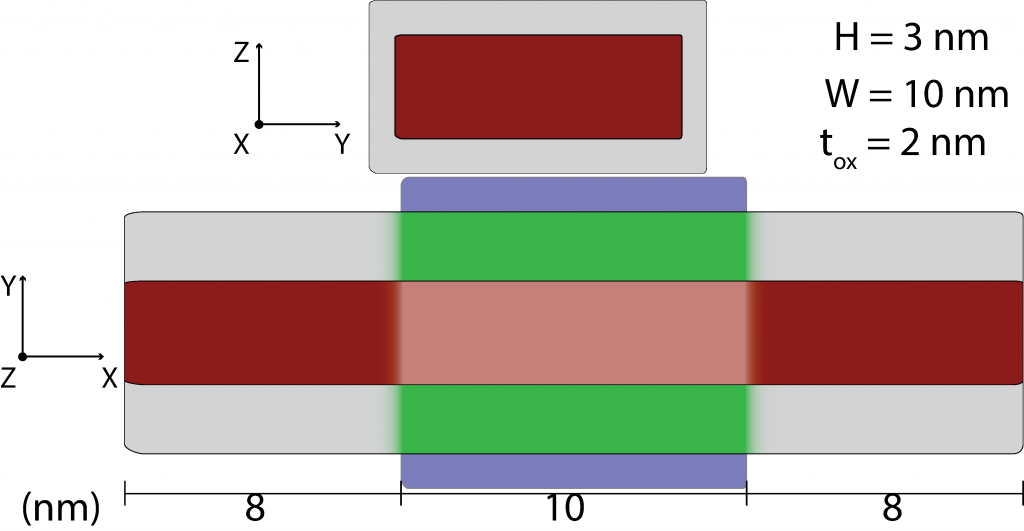Figure 1:In0.53Ga0.47As nanosheet FET: Schematic showing the device geometry. Red represents In0.53Ga0.47As, gray represents a low-κ oxide, green a high-κ oxide, and blue the gate electrode. In this tutorial we simulate a nanosheet-based device comprised of a III-V channel material. Nanosheet device types allow simulating the properties of quasi-planar devices where effects due to the semiconductor’s finite width are explicitly treated. To set up this simulation start a new M* workspace and set input parameters as:
1. Device select Double-gate nanosheet as the device type and enter geometrical parameters in accordance with figure 1 and as shown below in figure 2. Once you have finished, check the geometry corresponds to the intended design by visualising it in the Preview tab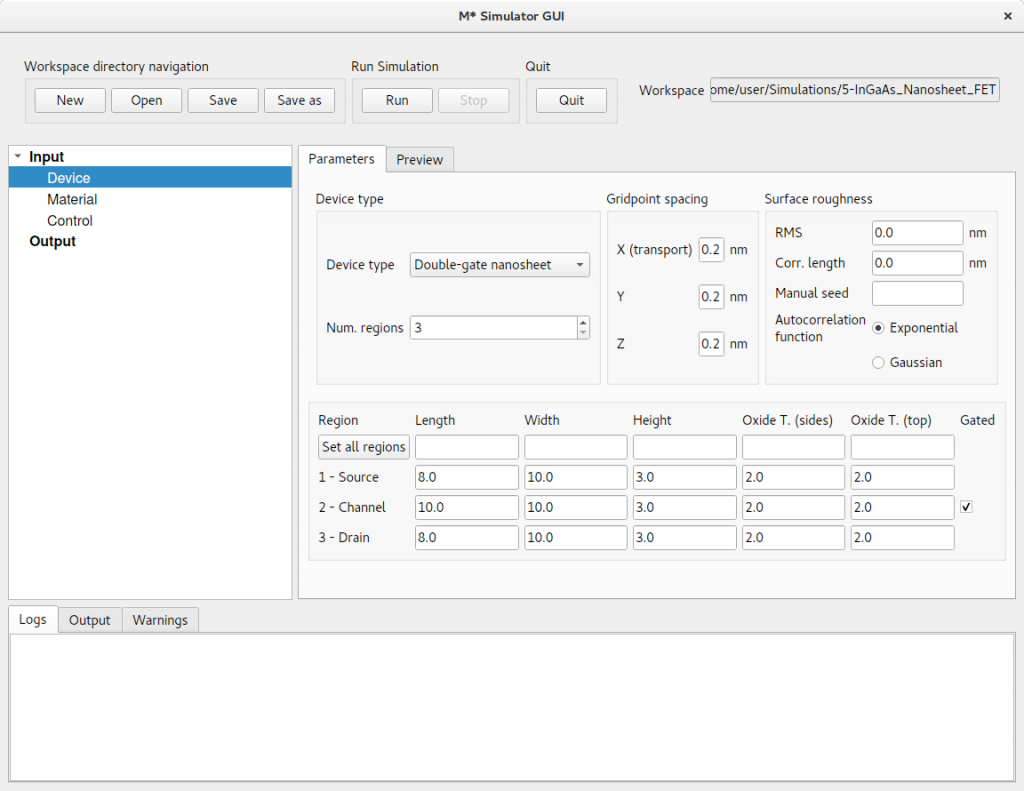Figure 2:In the Device panel, specify a Double-gate nanosheet with 3 regions and dimensions shown above.
2. Material select In(0.53)Ga(0.47)As, (010)/〈100〉, n-type and cycle through regions to set a target carrier concentration of 5 × 1019 cm-3 in source and drain extensions (i.e. regions 1 & 3), and zero in region 3. Input an oxide relative permittivity of εr = 4.0 (low-κ oxide) in source and drain extensions, and a value εr = 20.0 (high-κ oxide) in the channel. The low-κ oxide will reduce capacitive coupling between the gate electrode and source/drain extension regions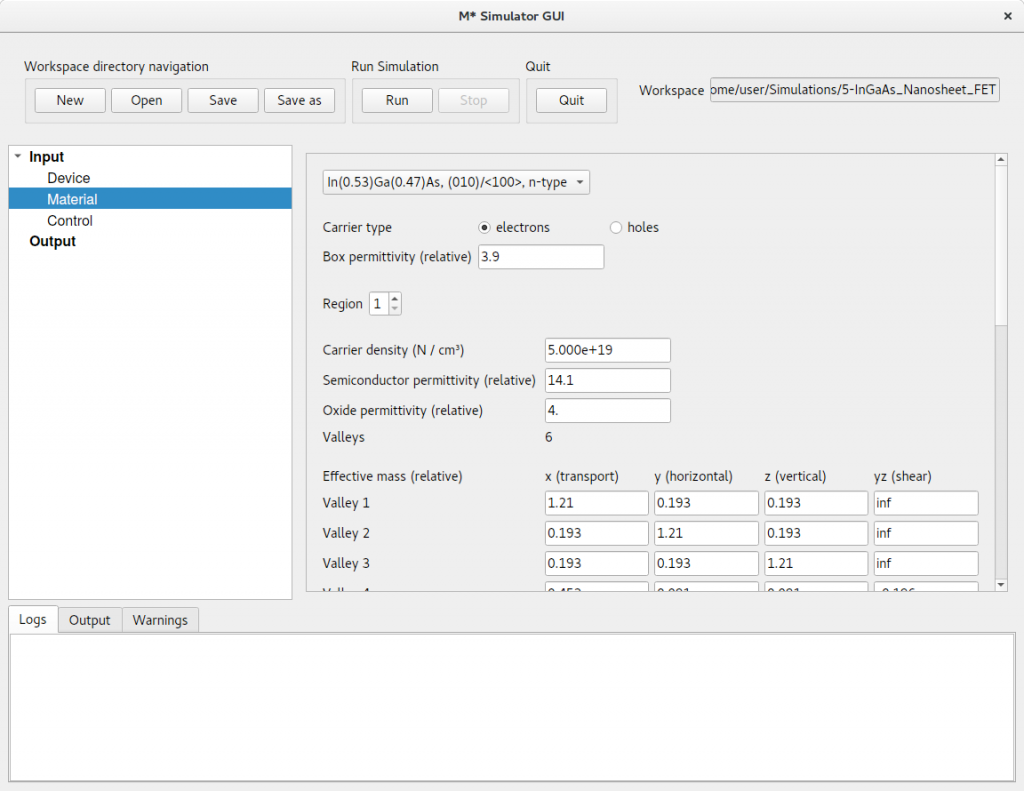Figure 3:In the Material panel, specify a carrier concentration of 5 × 1019 cm-3 and an oxide relative permittivity εr = 4.0 in both source and drain extensions, and a zero carrier concentration and &epsilonr = 20.0 in the channel.
3. Control select fast uncoupled-mode space (FUMS) as the mode-space method, and subbands to 6. Set a gate voltage sweep covering the [0.0,0.85] V range in steps of 0.05 V, and a drain voltage of 0.05 V. Enable saving electric potentials and carrier densities and set Save every  bias points to reduce disk space usage. Set the same convergence parameters as in previous tutorials and shown in figure 4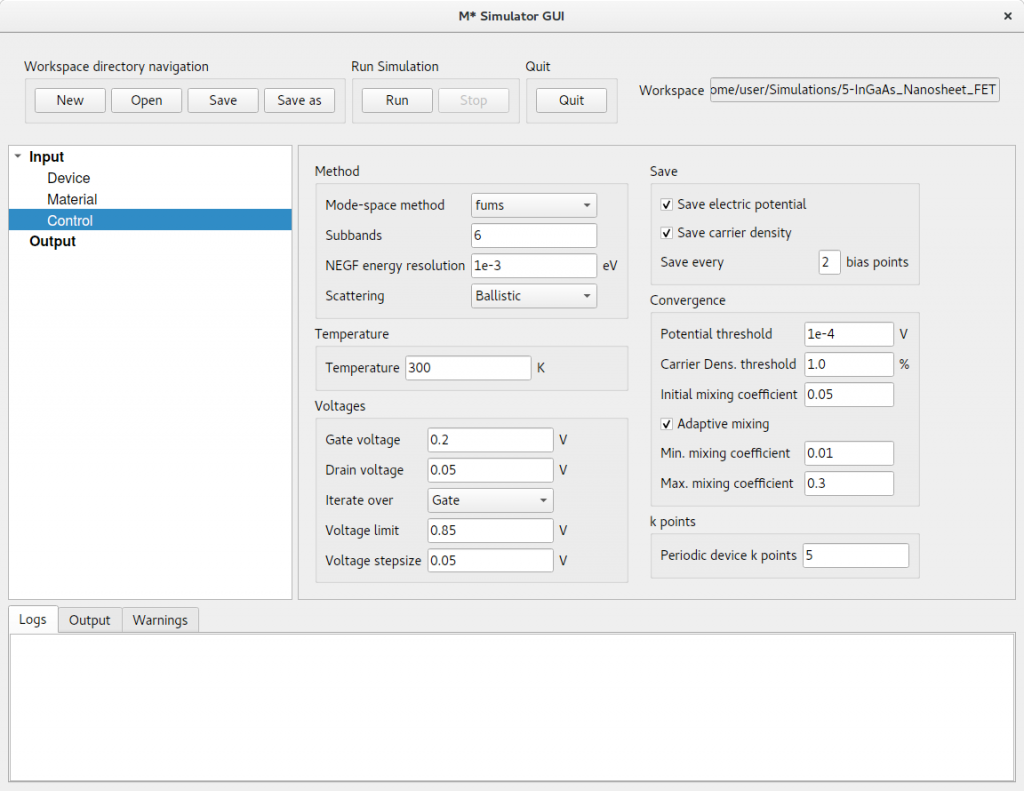Figure 4:In the Control panel, enter parameter values shown in the figure and discussed in the text. Click Run to begin the simulation. Once completed, you may visualise the 3D quantities to observe the influence of the device’s finite width on both electric potential and carrier density, as shown in figures 5 and 6 for an ON state.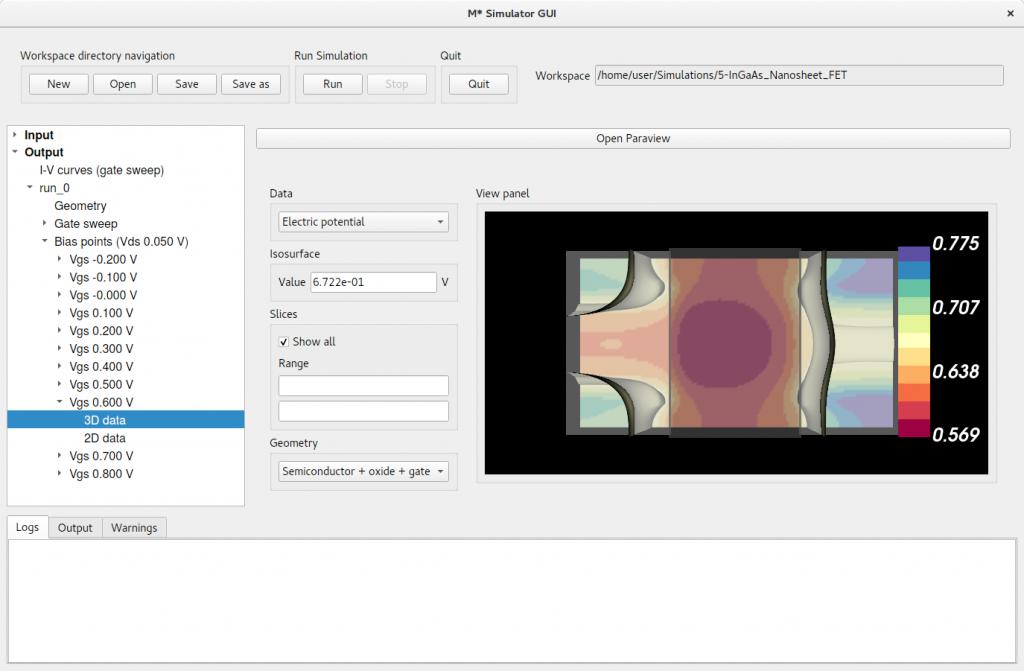Figure 5:Electric potential at VGS = 0.6 V (ON state).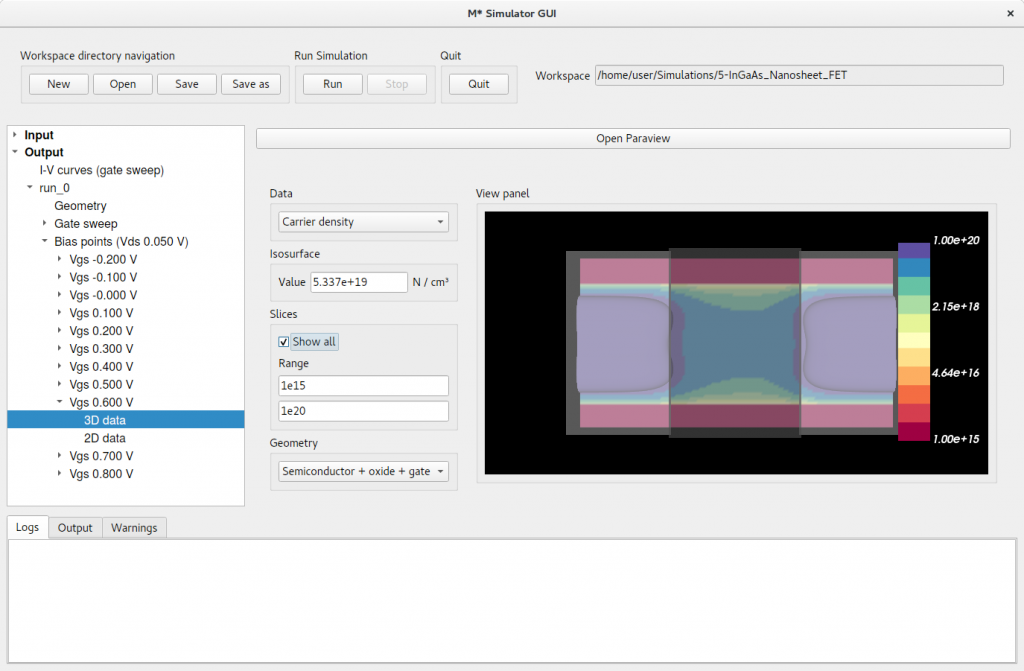Figure 6:Carrier density at VGS = 0.6 V (ON state). Note the range for the contour plot has been manually set to [1015,1020] cm-3 Next, inspect 2D data for various bias point to visualise plots similar to figures 7 and 8 (OFF and ON states, respectively). We can observe that the properties of this device are dominated by valleys 1 – 3, corresponding to the three X valleys in In0.53Ga0.47As. The significantly lower effective masses of valleys 4 & 5 (L valleys), and valley 6 (Γ valley) along cross-sectional dimensions result in larger confinement-induced shifts to their associated subbands towards higher energies, rendering their impact on the properties of this device negligible.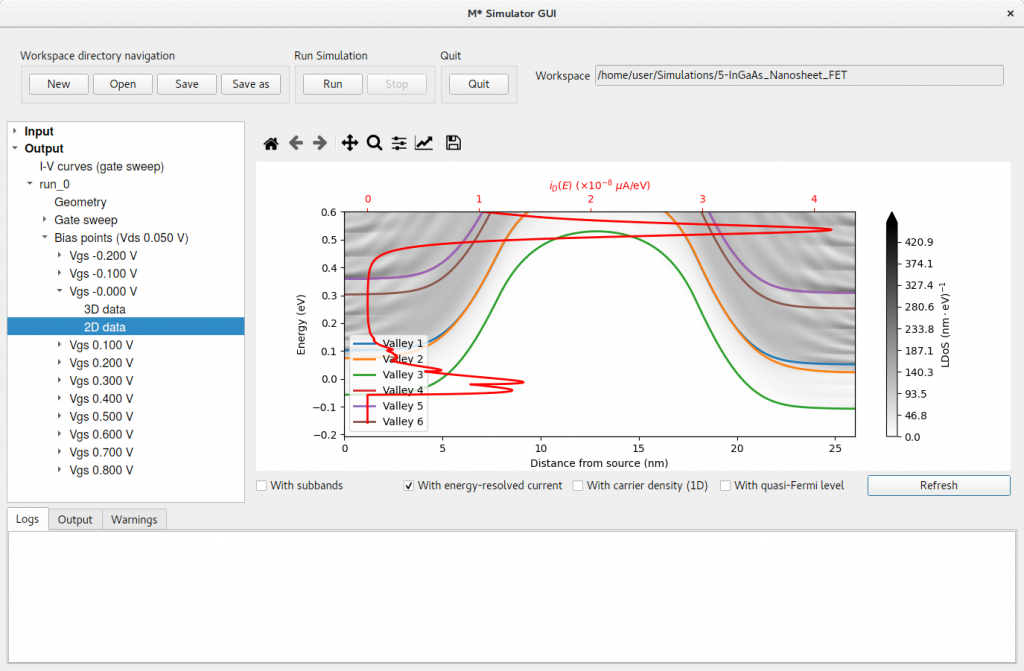Figure 7:Local density of states, lowest subband for each valley, and energy-resolved current at VGS = 0.0 V (OFF state).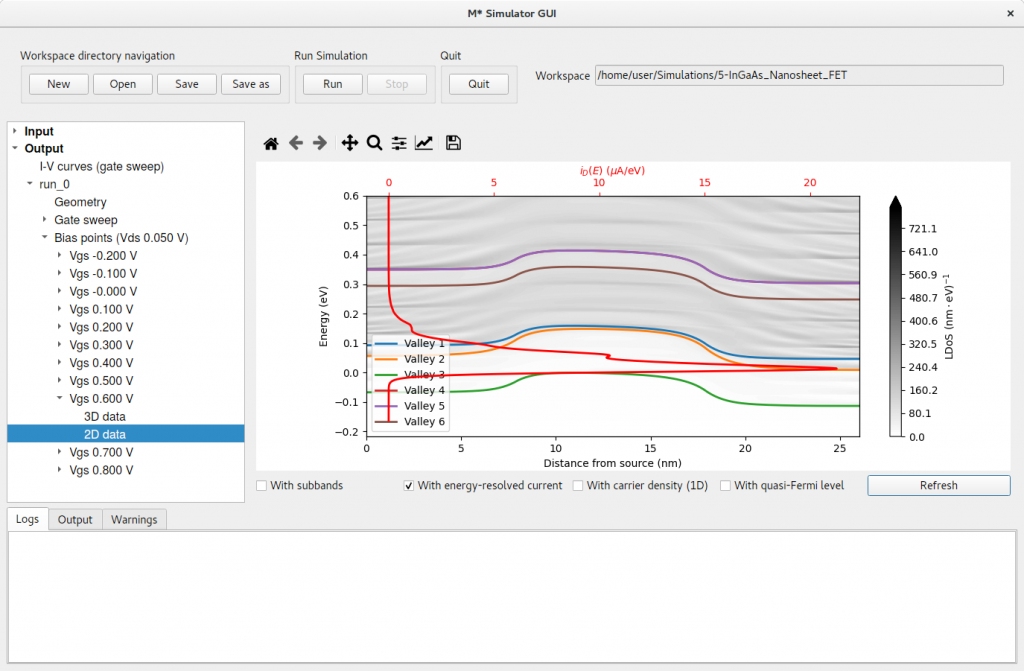Figure 8:Local density of states, lowest subband for each valley, and energy-resolved current at VGS = 0.6 V (ON state). To explore the geometrical dependence of confinement-induced energy shifts and their impact on device operation, let us simulate a similar device with a thicker channel:
1. Device edit the device geometry by modifying the value of Height fields with a value of 6 nm for all three regions
2. Control edit the gate voltage sweep range to [-0.2,0.85] V to cover more OFF states